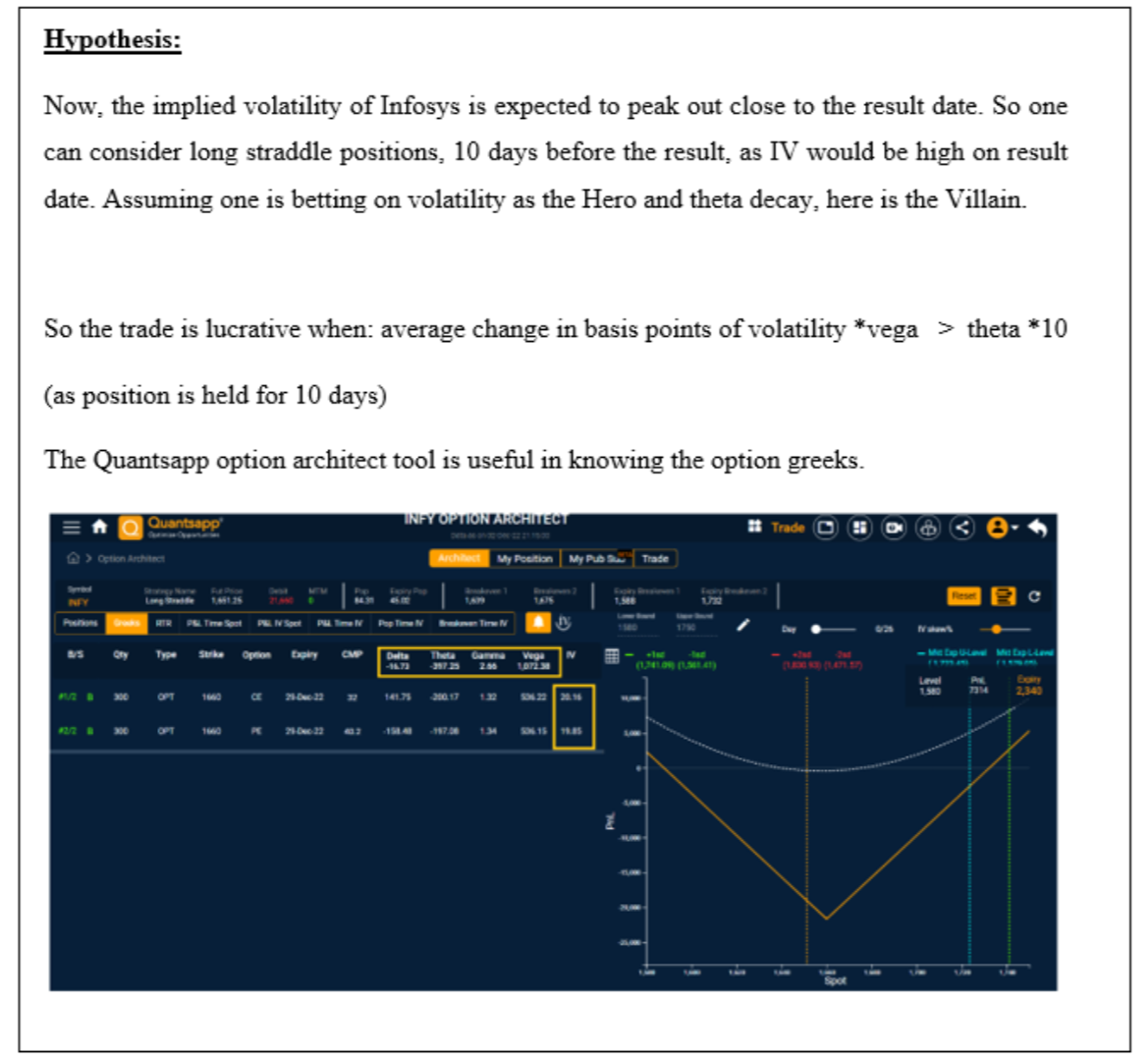Future realized volatility + Volatility tradingFuture realized volatility + Volatility trading

# Future realized volatility + Volatility trading

### What is future realized volatility?

We have studied historical volatility and implied volatility in past chapters, with the latter finding applications in options trading; as IV is forecasted volatility, with its value determined from the market price of options, using Black-Scholes option pricing model. While IV is the forecasted volatility, which is “expected” to prevail as we step into the future. But we are aware, that this is an estimate and the actual realized volatility during the forward-looking period may be different. This actual volatility observed during the forward-looking period is called future realized volatility (FRV).

The above exhibit shows the Nifty in orange colour, while FRV is indicated in blue colour. The computation of 1 Nov 2022 involves standard deviation of dataset of 20 days after the aforementioned date, which would give the FRV.

So,

Historical volatility (HV) 🔽                                           Volatility that was observed in the past

Implied volatility (IV) 🔽                                                A forecasted volatility that is derived from the  market price of options

Future realized volatility (FRV) 🔽                               Actual realized volatility which exists from the day of analysis to the required period in the future

### Historical volatility vs Future Realized Volatility

The above exhibit shows the comparison of HV and FRV for Nifty.

As we are aware, for a particular 20-day period, the volatility on day 1, taking into account the next 20 days is FRV. While considering the same time period, the volatility on day 20, taking into account the prior days to day 1 is HV. So, FRV today, is same has HV, 20 days later. Hence you observe that FRV data series leads by 20 days, as compared to HV.

### Result times and its tryst with volatility

Consider the example of INFOSYS and its result – dates, which are highlighted with dots on the IV curve in Quantsapp IV suite.### How do you calculate future realized volatility?

The future realized volatility is the standard deviation of returns or prices observed during the forward-looking period is called future realized volatility (FRV).

### Does implied volatility predict realized volatility?

Implied volatility is a forecast of future volatility, but what actually occurs is called future realized volatility or it’s the volatility which was realized during that forward looking period.

### What is the difference between implied and realized volatility?

Implied volatility is a forecast of future volatility arrived at from the market prices of options using Black Scholes option pricing model, but what actually occurs is called future realized volatility or it’s the volatility which was realized during that forward looking period.

### How do you trade volatility?

As volatility is expected to be mean-reverting, a period of elevated volatility could be followed by shrinkage or drop in volatility and vice versa. This characteristic needs to be exploited to develop long vol or short vol option trading strategies.

### How do you profit from volatility?

When volatility is expected to jump or increase, traders usually opt for long straddles/strangles, while if volatility is expected to shrink or reduce from elevated levels, traders sell options like short straddle or strangle and hedge by buying OTM puts and calls.